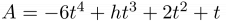## Problem J2: Who Has Seen The Wind

Margaret has looked at the wind floating over the prairies for a long time. After these observations, she has created a formula that will describe the altitude of a weather balloon launched from her house. In particular, her equation predicts the altitude A (in metres above the ground) at hour t after launching her balloon is:$A = -6t^4 + ht^3 + 2t^2 + t$

where h is an integer value representing the humidity as a value between 0 and 100 inclusive.

Margaret is curious at what the earliest hour is (if any) that her weather balloon will hit the ground after launch, so long as it is no more than the maximum time, M, that Margaret is willing to wait. You can assume that the weather balloon touches ground when A ≤ 0.

### Input Format

The input is two non-negative integers: h (0 ≤ h ≤ 100), the humidity factor, followed by M (0 < M < 240), the maximum number of hours Margaret will wait for the weather balloon to return to ground.

### Output Format

The output will be one of the following possibilities:

• `The balloon does not touch ground in the given time.`
• ```The balloon first touches ground at hour: T```

where T is a positive integer value representing the earliest hour when the balloon has altitude less than or equal to zero.

```30
10```

### Output

```The balloon first touches ground at hour:
6```

```70
10```

### Output

`The balloon does not touch ground in the given time.`

Point Value: 5
Time Limit: 2.00s
Memory Limit: 16M